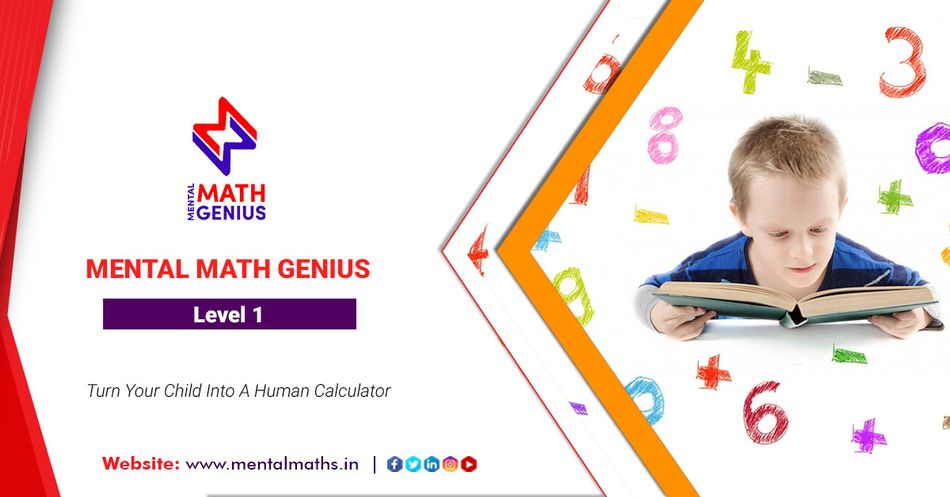### Mental Math Genius - (Level 1)

Share
₹ 7,500
Description

COURSE CONTENTS:

Mental Math Genius Level - 1
Learn to add, subtract, divide 3 digits mentally

2 Digit + 2 Digit
3 Digit + 3 Digit

QUICK SUBTRACTION
2 Digit - 2 Digit
3 Digit - 3 Digit

FAST DIVISION
Dividing by a Single Digit Divisor
Dividing by a Double-Digit Divisor
Dealing With 2 Digit Dividend
Dealing With 3 Digit Dividend

Missing Minuend
Adding / Subtracting Multiples of 10
Subtracting Across Zeroes
Rounding & Estimation
Place Value Concepts
Number Patterns
Comprehending Word Problems